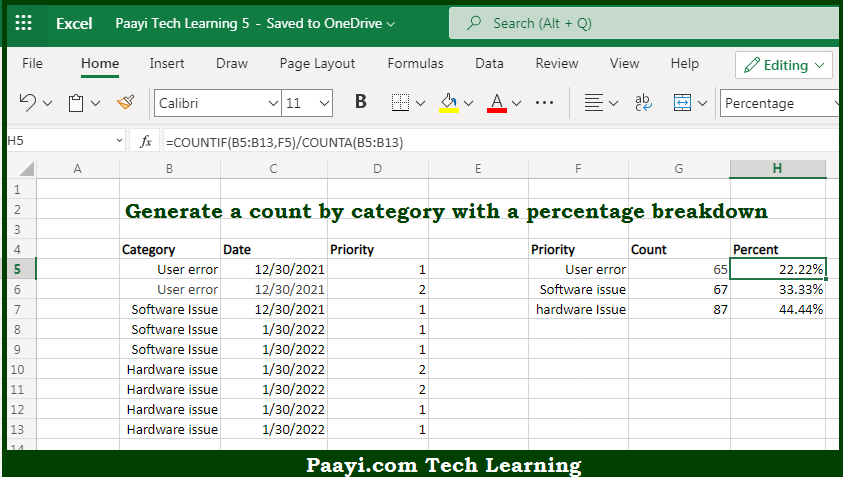# Learn How to Create Summary COUNT With Percentage Breakdown in Microsoft Excel

Written by | 0 Comments | 617 Views

In this article, you will learn how to COUNT various things in Microsoft Excel using a single or combination of functions and its purpose. You will also get to know how to Create a Summary COUNT With Percentage Breakdown and see the generic formula.

Create Summary COUNT With Percentage Breakdown in Microsoft Excel

The main purpose of this formula is to generate a count by category with a percentage breakdown. Here we will learn how to create a summary count with a percentage breakdown in Microsoft Excel. That implies, with the help of a formula based on the COUNTIF and COUNTA function you can able to generate a count by category with a percentage breakdown. So, with the help of this formula, you can able to create a summary count with a percentage breakdown.

General Formula to Create Summary COUNT With Percentage Breakdown

=COUNTIF(range,criteria)/COUNTA(range)

The Explanation for the Create Summary COUNT With Percentage BreakdownSo we know that with the help of the given formula above you can able to generate a count by category with a percentage breakdown. Here we will learn how to create a summary count with a percentage breakdown in Microsoft Excel. As we know that The range is the named range category as shown in the example.  The criteria as are supplied as a reference to the reference cell, which simply picks up the text value in a column. As the formula is copied down, it returns the count of each category listed in the F column. To calculate the percentage shown in column H, we need to divide the count per category by the total count. So, with the help of this formula, you can able to generate a count by category with a percentage breakdown.